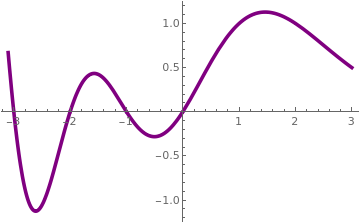# Preface

The gamma function Γ(x) is the natural extension of the factorial function $$n! = \prod_{k=1}^n k = 1 \cdot 2 \cdot 3 \cdots n$$ from integer n to real or complex x. It was first defined and studied by L. Euler in 18th century, who used the notation Γ(z), the capital letter gamma from the Greek alphabet. It is commonly used in many mathematical problems, including differential equations, but it appears occasionally in physical problems such as the normalization of Coulomb wave functions and the computation of probabilities in statistical mechanics. In general, however, it has less direct physical application and interpretation than, say, the Chebyshev and Bessel functions. Rather, its importance stems from its usefulness in developing other functions that have direct physical application.

Introduction to Linear Algebra with Mathematica

# Gamma Function of Euler

The first definition, given by Leonhard Euler is
$\Gamma (z) = \lim_{n\to \infty} \,\frac{n!}{z\left( z+1 \right) \left( z+2 \right) \cdots \left( z+n \right)} , \qquad z\ne 0, -1, -2, \ldots .$
This definition is not very useful in differential equations. The more common way to define the gamma function is through the following integral:
\begin{equation} \Gamma (\nu ) = \int_0^{\infty} t^{\nu -1} e^{-t} {\text d} t , \qquad \Re (\nu ) > 0. \label{EqGamma.1} \end{equation}
The restriction on ν is necessary to avoid divergence of the integral. There are known several equivalent definitions of the gamma function:
\begin{equation} \Gamma (\nu ) = 2 \int_0^{\infty} t^{2\nu -1} e^{-t^2} {\text d} t = \int_0^1 \left[ \ln \left( \frac{1}{t} \right) \right]^{\nu -1} {\text d} t . \label{EqGamma.2} \end{equation}Mathematica has a dedicated command for the gamma function -- Gamma[ z ]. You can make a fancy plotof this function: Plot[Gamma[x], {x, -3, 3}, PlotStyle -> {Purple, Thickness[0.01]}] The gamma function has a local minimum at +1.46163214496836234126. The Gamma function. Mathematica code

When ν = ½, Eq.\eqref{EqGamma.1} is just the Gauss erroe integral $$\Gamma (1/2) = \sqrt{\pi} .$$ In general, we have
\begin{equation} \Gamma \left( \frac{1}{2} + n \right) = \frac{(2n)!}{4^n n!}\,\sqrt{\pi} = \frac{(2n-1)!!}{2^n} \,\sqrt{\pi} \qquad\mbox{and} \qquad \Gamma \left( \frac{1}{2} - n \right) = \frac{(-4)^n n!}{(2n)!}\,\sqrt{\pi} = \frac{(-2)^n}{(2n-1)!!} \,\sqrt{\pi} . \label{EqGamma.3} \end{equation}
One of the most important properties of the gamma function is its recurrence relation (difference equation of the first order)
\begin{equation} \Gamma (\nu +1) = \nu\,\Gamma (\nu ) , \qquad \Gamma (1) = \Gamma (2) = 1. \label{EqGamma.4} \end{equation}
This leads to the remarkable formula that generalizes the factorial:
\begin{equation} \Gamma (n +1) = 1 \cdot 2 \cdot 2 \cdots n = n! \qquad\mbox{for integer} \quad n \ge 0. \label{EqGamma.5} \end{equation}
Other important functional equations for the gamma function are Euler's reflection formula
\begin{equation} \Gamma \left( 1-z \right) \Gamma (z) = \frac{\pi}{\sin (\pi z)} , \qquad z \notin \mathbb{Z} . \label{EqGamma.6} \end{equation}With Mathematica, one can plot the reciprocal of the gamma functions: Plot[1/Gamma[x], {x, -3.1, 3}, PlotStyle -> {Purple, Thickness[0.01]}] The reciprocal of the Gamma function. Mathematica code

Incomplete gamma function

The upper incomplete gamma function is defined as:
$\Gamma (\nu , x) = \int_x^{\infty} t^{\nu -1} e^{-t} {\text d} t ,$
whereas the lower incomplete gamma function is defined as:
$\gamma (\nu , x) = \int_0^x t^{\nu -1} e^{-t} {\text d} t .$
Clearly, the two functions are related, for
$\gamma (\nu , x) + \Gamma (\nu , x) = \Gamma (\nu ) .$

Beta function

The gamma function is related to the beta function by the formula
$B(x,y) = \int_0^1 t^{x-1} \left( 1- t \right)^{y-1} {\text d} t = \frac{\Gamma (x)\,\Gamma (y)}{\Gamma (x+y)} .$

Digamma function

the digamma function is defined as the logarithmic derivative of the gamma function
$\psi (x) = \frac{\text d}{{\text d}x} \, \ln \left( \Gamma (x) \right) = \frac{\Gamma' (x)}{\Gamma (x)} .$
Then the derivatives of the gamma function are described in terms of the polygamma function
$\Gamma' (z) = \Gamma (z)\,\psi_0 (z)$
The recurrence relation for the digamma function is
$\psi (z+1) = \psi (x) + \frac{1}{z} .$
This allows to relate the digamma function for integers and the the harmonic numbers:
$H_n = 1 + \frac{1}{2} + \cdots + \frac{1}{n} = \sum_{k=1}^n \frac{1}{k} = \psi (n+1) + \gamma ,$
where γ = 0.57721566490153286060651209008240243104215933593992... is the Euler constant (also known as Euler–Mascheroni constant).

The exponential integral

the exponential integral Ei is a special function on the complex plane ℂ:
$\mbox{Ei}(z) = - \int_{-x}^{\infty} \frac{e^{-t}}{t}\,{\text d} t = \int_{-\infty}^x \frac{e^{t}}{t}\,{\text d} t$